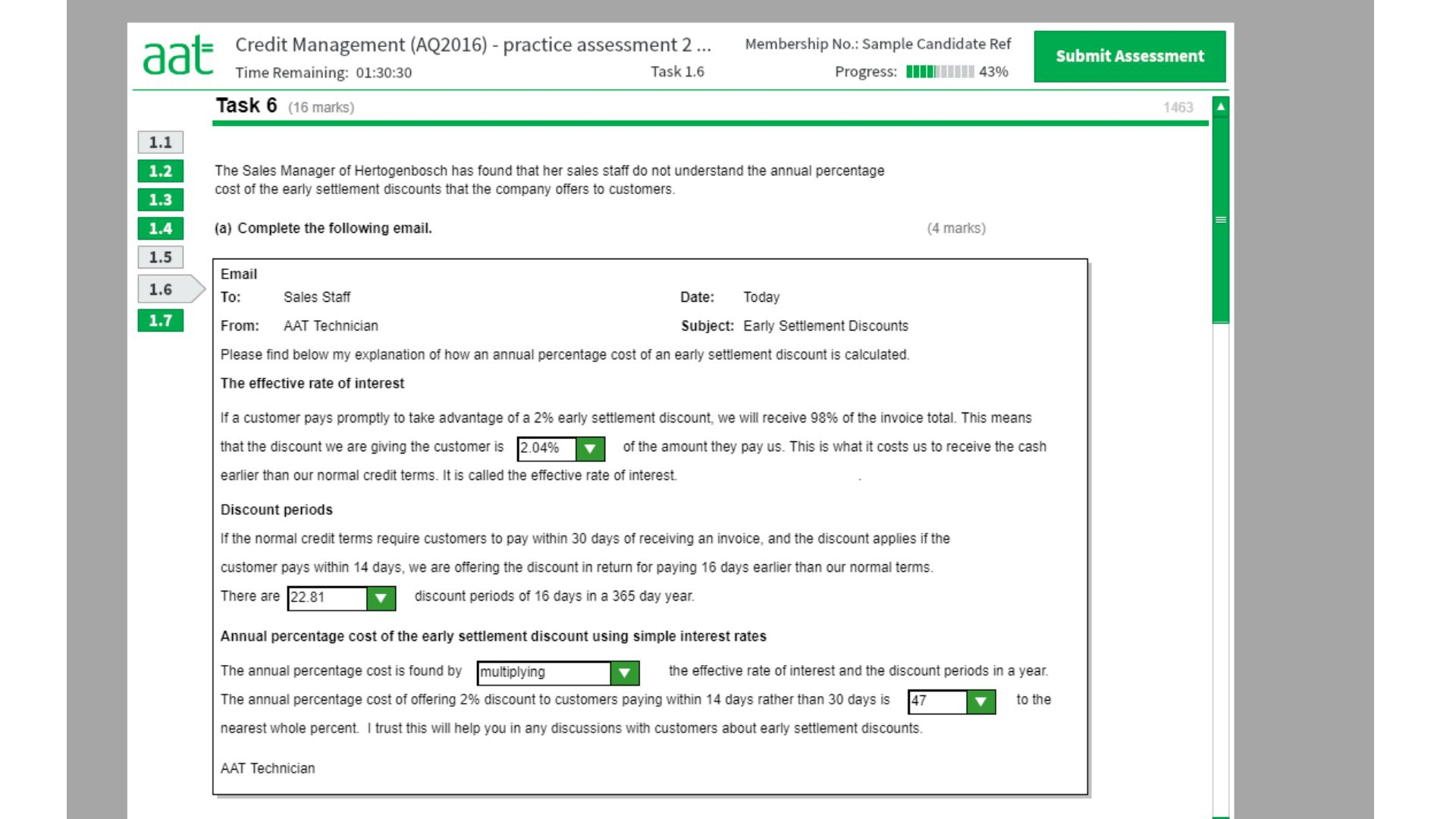# Practice asessment 2 task 6 Credit management, does anyone know how to calculate this please?• Effective rate interest

Formula= d/100-d X 365/N-D

d= discount percentage given
N=normal payment term
D=Discount payment term

Therefore we do not have any days given so we will only use the percentage side

2%/98% *100 =2.04%

discounts periods

Using the same method

Here we do not have the percentage side so it is going to be a straight

365/16 =22.81

Finally

2.04% X 22.81 =46.53

=47%

OR

2%/100%-2% X 365/30-14

2/98 X 365/16

0.02040816326 X 22.8125

0.4655 *100%

=47%